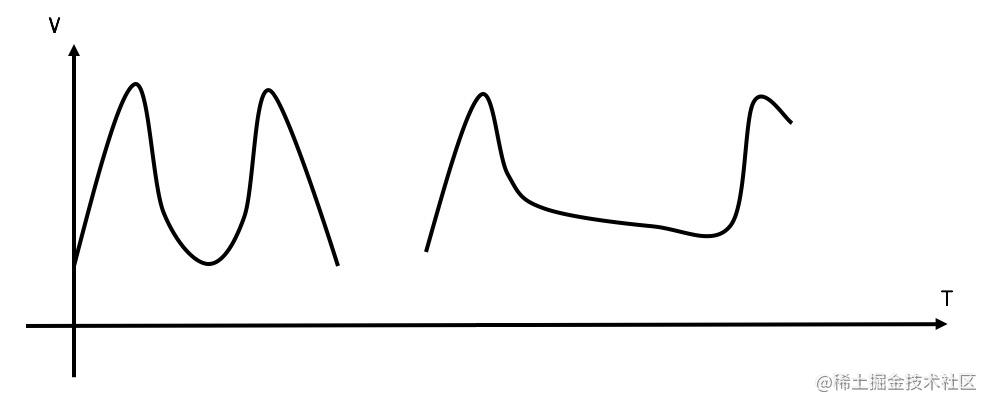# 接口限流算法总结

## 算法介绍

### 计数器法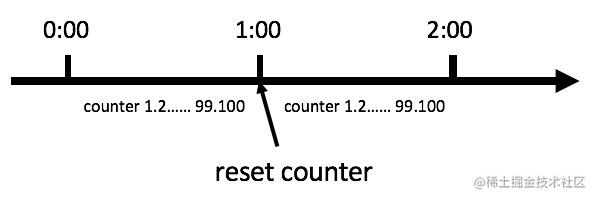``````public class CounterDemo {
public long timeStamp = getNowTime();
public int reqCount = 0;
public final int limit = 100; // 时间窗口内最大请求数
public final long interval = 1000; // 时间窗口ms
public boolean grant() {
long now = getNowTime();
if (now < timeStamp + interval) {
// 在时间窗口内
reqCount++;
// 判断当前时间窗口内是否超过最大请求控制数
return reqCount > limit;
}
else {
timeStamp = now;
// 超时后重置
reqCount = 1;
return true;
}
}
}复制代码``````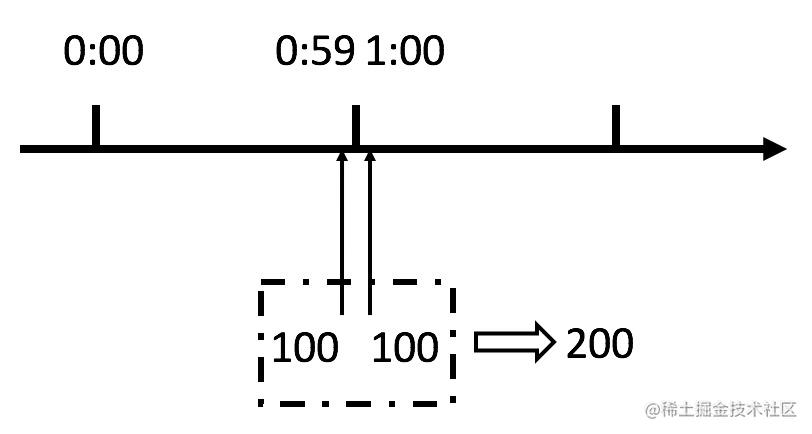### 滑动窗口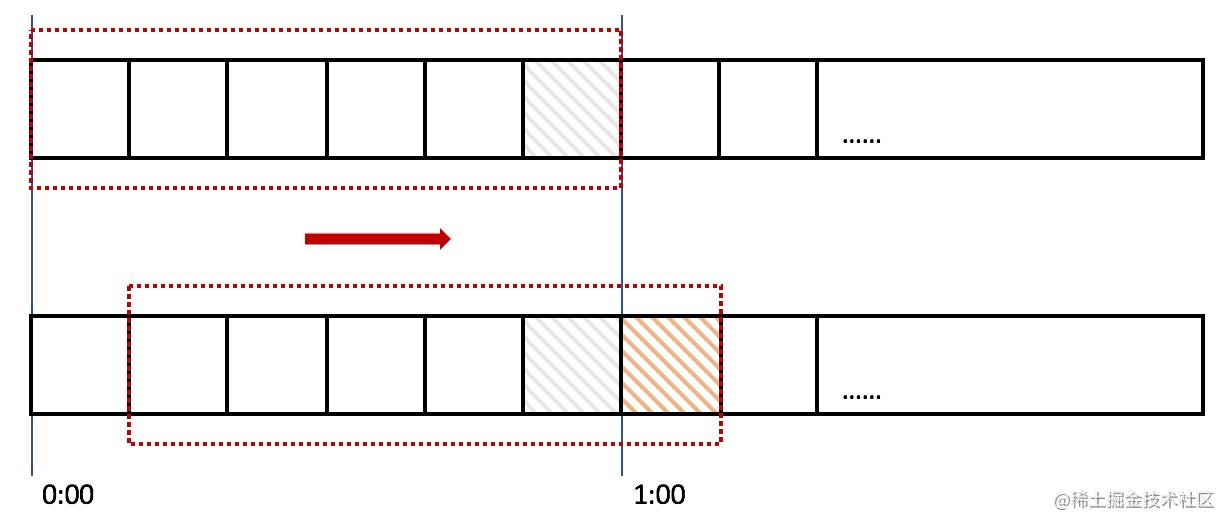### 漏桶算法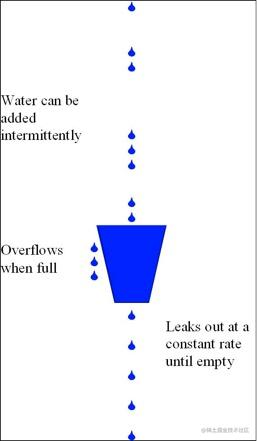``````public class LeakyDemo {
public long timeStamp = getNowTime();
public int capacity; // 桶的容量
public int rate; // 水漏出的速度
public int water; // 当前水量(当前累积请求数)
public boolean grant() {
long now = getNowTime();
water = max(0, water - (now - timeStamp) * rate); // 先执行漏水，计算剩余水量
timeStamp = now;
if ((water + 1) < capacity) {
// 尝试加水,并且水还未满
water += 1;
return true;
}
else {
// 水满，拒绝加水
return false;
}
}
}复制代码``````

### 令牌桶算法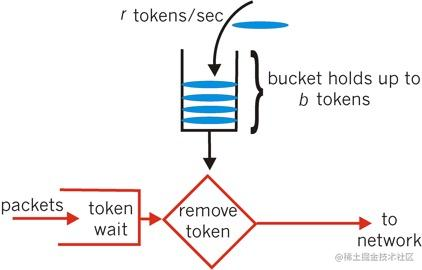``````public class TokenBucketDemo {
public long timeStamp = getNowTime();
public int capacity; // 桶的容量
public int rate; // 令牌放入速度
public int tokens; // 当前令牌数量
public boolean grant() {
long now = getNowTime();
// 先添加令牌
tokens = min(capacity, tokens + (now - timeStamp) * rate);
timeStamp = now;
if (tokens < 1) {
// 若不到1个令牌,则拒绝
return false;
}
else {
// 还有令牌，领取令牌
tokens -= 1;
return true;
}
}
}复制代码``````

#### 临界问题